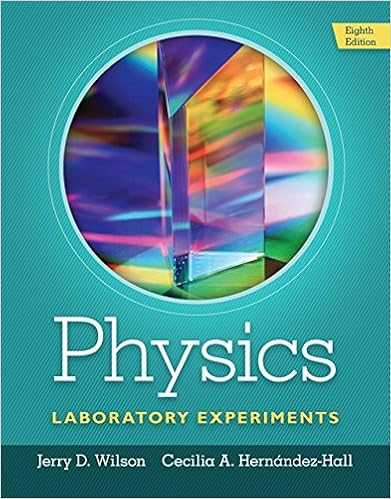# The relationship between fsmax and the normal force

• Lab Report
• 6
• 100% (4) 4 out of 4 people found this document helpful

This preview shows page 1 - 3 out of 6 pages.

##### We have textbook solutions for you!
The document you are viewing contains questions related to this textbook.The document you are viewing contains questions related to this textbook.
Chapter 11 / Exercise 1
Physics Laboratory Experiments
WilsonExpert Verified
The relationship between fsmax and the normal force FN is 2. fs,maxsFN, where μsis a constant, called the coefficient of static friction. Once the object begins to slide, the kinetic friction will take over the static friction. The kinetic friction will take over the static friction. The kinetic frictional force between the two surfaces is given by: 3. fkkFN, where k is the coefficient of kinetic (sliding) friction If the sliding takes place at the constant speed, Newton’s First Law can be applied to the system.
##### We have textbook solutions for you!
The document you are viewing contains questions related to this textbook.The document you are viewing contains questions related to this textbook.
Chapter 11 / Exercise 1
Physics Laboratory Experiments
WilsonExpert Verified
Both static and kinetic friction coefficients depend only on the nature of the two surfaces in contact with each other, and are independent of the normal force exerted between them. The coefficient of kinetic friction is typically smaller than the coefficient of static friction, reflecting the common experience that is easier to keep something in motion across a horizontal surface than to start it in motion from rest. Equations 2 or 3 is a linear dependence of frictional vs. normal force. The slopes of the regression line should be the corresponding coefficients of static and kinetic friction. Your experimental points should follow a straight line. EXPERIMENTAL PROCEDURE:1.Log into the computer using your UCID and password. 2.Connect the USB cable of the 850 Universal Interface to a USB port on the computer. 3.Connect the AC adapter power cord to an electrical outlet under the lab table. 4.Press the power push button on the left corner of the interface. The green LED indicator below the power push button should light up. 5.Open “Lab 106 Friction” file in “Physics 102A Lab Experiments” folder on Desktop. You will see “Measurement”, “Analysis1” and “Analysis2” pages on the screen. 6.Measure the mass of the Friction Tray. Record the values. 7.Plug the Force Sensor to “PASPort2” input and press “Zero” button on the sensor with no force applied. 8.Put a mass in the Friction Tray. Connect the Friction Tray to the Force Sensor using the string. 9.Go to page of “Measurement” and click “RECORD” button on the bottom of the screen. 10. Place the Friction Tray on a lab table. With the force sensor tied to the tray, slowly pull the Friction Tray horizontally, from rest, across the table until it reaches a constant velocity. Continue pulling at a constant velocity.
•••